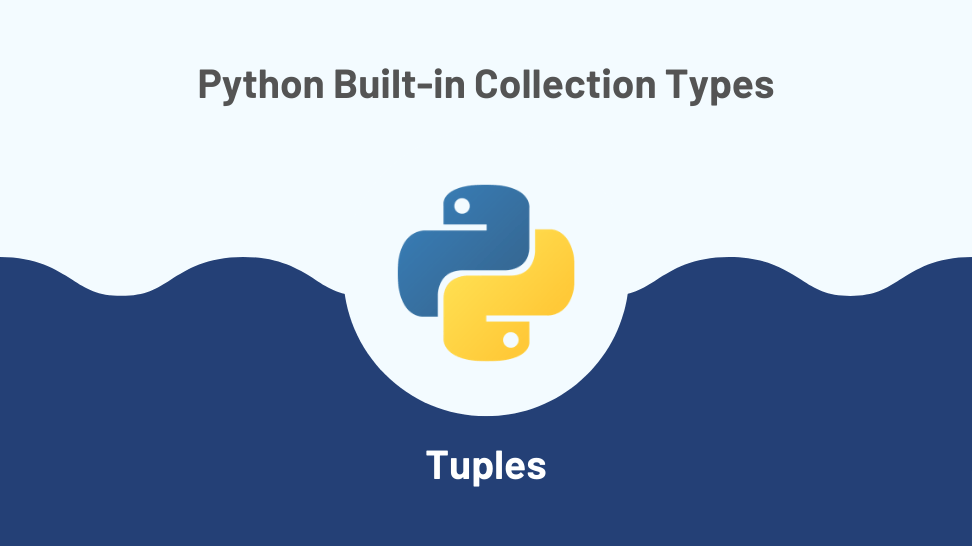In this article, I’ll explore the tuple, one of 4 built-in types used to store collections in Python.

This post is the second article in a miniseries exploring the topic of built-in collection types in Python. I based the series on my notes while studying for a Python technical interview.

For quick access, here is the list of posts on this series:

1. Python Lists
3. Python Sets
4. Python Dictionaries

## Introduction to tuples

In Python, a tuple is a data type used for storing a collection of objects. Tuples have the following characteristics:

### Tuples have an order

Tuples preserve the order of their elements. Two tuples with the same elements but in different order are not equal:

### Tuples can contain any arbitrary object

Tuple elements can be of any data type. The example below shows a tuple containing integers, floats, boolean, strings, dictionaries, and other tuple.

### Tuples can contain duplicates

The same element can exist at different indexes in a tuple. For example, the tuple below contains the integer 1 at positions 0 and 2.

### Tuples are immutable

You can’t change, add or remove elements after creating a tuple.

## Creating a tuple

You can create a tuple using a tuple literal by wrapping its elements in round brackets:

When creating a tuple with round brackets, if the tuple has only one element, you should use a trailing comma:

You can also use the tuple () constructor:

## Accessing elements from a tuple

You can access an element from a tuple by using the element index. The index indicates the position of the element within the tuple. Indexes in sequential data types in Python start at 0.

## Tuple slicing

By slicing a tuple, you can obtain a new tuple that is a sub-tuple of the original. The syntax for slicing is:

Which would return a sub-tuple from index = start to index = end -1 using the given step (or 1 if no step is given).

Let’s look a some examples.

## Tuple built-in methods

### tuple.index(x)

The .index(x) method returns the index of the first occurrence of x in the tuple. This method has a linear time complexity of O(n), where n is the length of the tuple.

### tuple.count(x)

The .count(x) method returns the number of times x appears in the tuple. This method has a linear time complexity of O(n), where n is the length of the tuple.

## Tuple unpacking

As with other iterables in Python, you can unpack the elements of a tuple into individual variables in one line:

The only requirement is that the number of variables on the left of the assignment operator is equal to the length of the tuple.

## Lists or tuples?

So, what should you use, a list or a tuple? The short answer is to use a tuple if the values in the collection should remain constant over the program’s life. That way, you’ll enjoy faster execution and avoid bugs introduced by accidentally modifying the values in the collection.

## Conclusion

This article covered Python tuples, a built-in data type you will most likely use in any non-trivial coding project. We covered the main characteristics of tuples, the topic of slicing, the tuple built-in methods with their time complexity, and tuple unpacking.

We also gave a rule of thumb for when to use tuples instead of lists: always use tuples if the values in the collection should remain constant over the program’s life.